1
70
views
11a
Problem

# Problem 11a

## Chapter 1: Functions and ModelsTextbook ExpertVerified Tutor
14 Oct 2021

#### Given information

Given the equation for dosage as: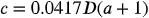and dosage for an adult as 200 mg

#### Step-by-step explanation

Step 1.

Now, in order to find the slop of the equation, the dosage of an adult is 200 is substituted in the equation of dosage.

The equation will look like -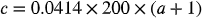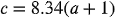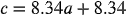Now, the equation has reached the form of equation -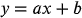, where a = slope of the equation and b is the intercept.

In the equation,, slope is 8.34 and it represents the change in mg for every 1 year change.